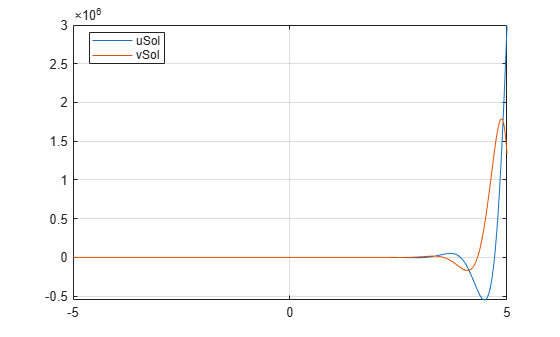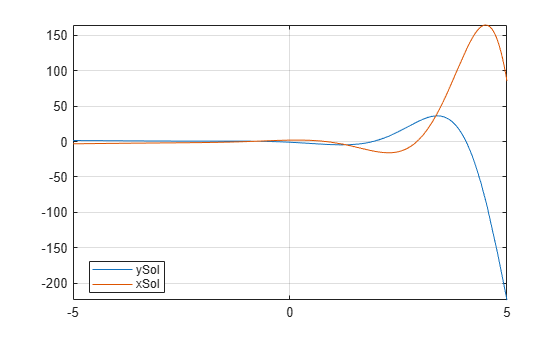# Solve a System of Differential Equations

Solve a system of several ordinary differential equations in several variables by using the `dsolve` function, with or without initial conditions. To solve a single differential equation, see Solve Differential Equation.

### Solve System of Differential Equations

Solve this system of linear first-order differential equations.

`$\begin{array}{l}\frac{\mathit{du}}{\mathit{dt}}=3\mathit{u}+4\mathit{v},\\ \frac{\mathit{dv}}{\mathit{dt}}=-4\mathit{u}+3\mathit{v}.\end{array}$`

First, represent $u$ and $v$ by using `syms` to create the symbolic functions `u(t)` and `v(t)`.

`syms u(t) v(t)`

Define the equations using `==` and represent differentiation using the `diff` function.

```ode1 = diff(u) == 3*u + 4*v; ode2 = diff(v) == -4*u + 3*v; odes = [ode1; ode2]```
```odes(t) =  ```

Solve the system using the `dsolve` function which returns the solutions as elements of a structure.

`S = dsolve(odes)`
```S = struct with fields: v: C1*cos(4*t)*exp(3*t) - C2*sin(4*t)*exp(3*t) u: C2*cos(4*t)*exp(3*t) + C1*sin(4*t)*exp(3*t) ```

If `dsolve` cannot solve your equation, then try solving the equation numerically. See Solve a Second-Order Differential Equation Numerically.

To access `u(t)` and `v(t)`, index into the structure `S`.

`uSol(t) = S.u`
`uSol(t) = ${C}_{2} \mathrm{cos}\left(4 t\right) {\mathrm{e}}^{3 t}+{C}_{1} \mathrm{sin}\left(4 t\right) {\mathrm{e}}^{3 t}$`
`vSol(t) = S.v`
`vSol(t) = ${C}_{1} \mathrm{cos}\left(4 t\right) {\mathrm{e}}^{3 t}-{C}_{2} \mathrm{sin}\left(4 t\right) {\mathrm{e}}^{3 t}$`

Alternatively, store `u(t)` and `v(t)` directly by providing multiple output arguments.

`[uSol(t),vSol(t)] = dsolve(odes)`
`uSol(t) = ${C}_{2} \mathrm{cos}\left(4 t\right) {\mathrm{e}}^{3 t}+{C}_{1} \mathrm{sin}\left(4 t\right) {\mathrm{e}}^{3 t}$`
`vSol(t) = ${C}_{1} \mathrm{cos}\left(4 t\right) {\mathrm{e}}^{3 t}-{C}_{2} \mathrm{sin}\left(4 t\right) {\mathrm{e}}^{3 t}$`

The constants `C1` and `C2` appear because no conditions are specified. Solve the system with the initial conditions `u(0) == 0` and `v(0) == 0`. The `dsolve` function finds values for the constants that satisfy these conditions.

```cond1 = u(0) == 0; cond2 = v(0) == 1; conds = [cond1; cond2]; [uSol(t),vSol(t)] = dsolve(odes,conds)```
`uSol(t) = $\mathrm{sin}\left(4 t\right) {\mathrm{e}}^{3 t}$`
`vSol(t) = $\mathrm{cos}\left(4 t\right) {\mathrm{e}}^{3 t}$`

Visualize the solution using `fplot`.

```fplot(uSol) hold on fplot(vSol) grid on legend('uSol','vSol','Location','best')```### Solve Differential Equations in Matrix Form

Solve differential equations in matrix form by using `dsolve`.

Consider this system of differential equations.

`$\begin{array}{l}\frac{\mathit{dx}}{\mathit{dt}}=\mathit{x}+2\mathit{y}+1,\\ \frac{\mathit{dy}}{\mathit{dt}}=-\mathit{x}+\mathit{y}+\mathit{t}.\end{array}$`

The matrix form of the system is

`$\left[\begin{array}{c}{\mathit{x}}^{\prime }\\ {\mathit{y}}^{\prime }\end{array}\right]=\left[\begin{array}{cc}1& 2\\ -1& 1\end{array}\right]\left[\begin{array}{c}\mathit{x}\\ \mathit{y}\end{array}\right]+\left[\begin{array}{c}1\\ \mathit{t}\end{array}\right].$`

Let

`$\mathit{Y}=\left[\begin{array}{c}\mathit{x}\\ \mathit{y}\end{array}\right],\mathit{A}=\left[\begin{array}{cc}1& 2\\ -1& 1\end{array}\right],\mathit{B}=\left[\begin{array}{c}1\\ \mathit{t}\end{array}\right].$`

The system is now ${\mathit{Y}}^{\prime }=\mathit{A}\text{\hspace{0.17em}}\mathit{Y}+\mathit{B}.$.

Define these matrices and the matrix equation.

```syms x(t) y(t) A = [1 2; -1 1]; B = [1; t]; Y = [x; y]; odes = diff(Y) == A*Y + B```
```odes(t) =  ```

Solve the matrix equation using `dsolve`. Simplify the solution by using the `simplify` function.

```[xSol(t),ySol(t)] = dsolve(odes); xSol(t) = simplify(xSol(t))```
```xSol(t) =  $\frac{2 t}{3}+\sqrt{2} {C}_{2} {\mathrm{e}}^{t} \mathrm{cos}\left(\sqrt{2} t\right)+\sqrt{2} {C}_{1} {\mathrm{e}}^{t} \mathrm{sin}\left(\sqrt{2} t\right)+\frac{1}{9}$```
`ySol(t) = simplify(ySol(t))`
```ySol(t) =  ${C}_{1} {\mathrm{e}}^{t} \mathrm{cos}\left(\sqrt{2} t\right)-\frac{t}{3}-{C}_{2} {\mathrm{e}}^{t} \mathrm{sin}\left(\sqrt{2} t\right)-\frac{2}{9}$```

The constants `C1` and `C2` appear because no conditions are specified.

Solve the system with the initial conditions $u\left(0\right)=2$ and $v\left(0\right)=-1$. When specifying equations in matrix form, you must specify initial conditions in matrix form too. `dsolve` finds values for the constants that satisfy these conditions.

```C = Y(0) == [2;-1]; [xSol(t),ySol(t)] = dsolve(odes,C)```
```xSol(t) =  ```
```ySol(t) =  ```

Visualize the solution using `fplot`.

```clf fplot(ySol) hold on fplot(xSol) grid on legend('ySol','xSol','Location','best')```